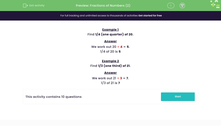# Find Fractions of Numbers

In this worksheet, students will find simple fractions of numbers by dividing.Key stage:  KS 1

Curriculum topic:   Number: Fractions

Curriculum subtopic:   Write Fractions

Popular topics:   Fractions worksheets

Difficulty level:#### Worksheet Overview

In this activity, we are going to find fractions of numbers.

To find a fraction of a whole number, we need to divide the whole number by the bottom number of the fraction (the denominator).

Example 1

Find 1/4 (one quarter) of 20.

We need to divide 20 by the bottom number, which is 4:

We work out 20 ÷ 4 = 5

1/4 of 20 is 5

Example 2

Find 1/3 (one third) of 21.

We need to divide 21 by the bottom number, which is 3:

We work out 21 ÷ 3 = 7

1/3 of 21 is 7

Example 3

Find 1/5 (one fifth) of 30.

We need to divide 30 by the bottom number, which is 5.

We work out 30  ÷  5 = 6

1/5 of 30 is 6.

Have you got the idea now? Let's try some questions.### What is EdPlace?

We're your National Curriculum aligned online education content provider helping each child succeed in English, maths and science from year 1 to GCSE. With an EdPlace account you’ll be able to track and measure progress, helping each child achieve their best. We build confidence and attainment by personalising each child’s learning at a level that suits them.

Get started